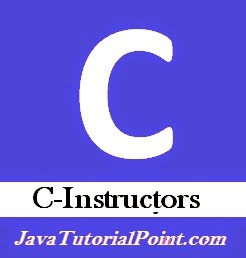## Pages

### 4. C-Instructors

C-Basic Programs: Previous                                                                  Next: C-Operators

Generally we have used three types of instruction in C Programming:1. Type declaration instructions.
2. Arithmetic instructions.
3. Control instructions.
1. Type Declaration: These are known as data types and are used to declare variables of a program. (Described Previously).
2. Arithmetic Instructions: These are used  to perform arithmetic operations on variables or constants. It consist the arithmetic operator, assignment operator and  variable or  constant on which operation can be performed. Constant can b e integer or real. The statement on which arithmetic instruction s are  performed is known as arithmetic expression.
Example :  float per;
int math,sci,so;
per= math +  sci + so/3;
Arithmetic expressions can be written in 3 ways :
a. Expression,  which consist all integer variables and constants.
Example :  int sum,a,b,c;
Sum =a*b*c;

b. Expression, which consist all float type variables and constant.
c. Expression, which consist mixed of both integer and float.
Example : float salary;
int bonus;
salary =salary+bonus;
3. Control instructions are used to take decision based on some conditions.

### Decision Control Instructions:

These are sequence or set of instruction which helps compiler to take decision according to different situations. All the statements which allows you to change the sequence of instructions are known as “Conditional Statements” or “Control Statements
Control statements are of two types:

1. Conditional Branching
2. Looping
Conditional Branching: -
Generally it is used to ignore the sequential branching in which we execute a statement one after another without skipping a single one. Conditional branching executes all the statements based on some conditions.
Generally there are two types of conditional branching:

1. if statement branching
2. if else statement branching
Before starting the actual control statements I would like you to make familiar with the operators used in C Programming.

C-Basic Programs: Previous                                                                  Next: C-Operators